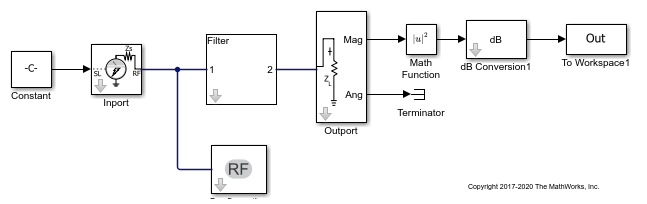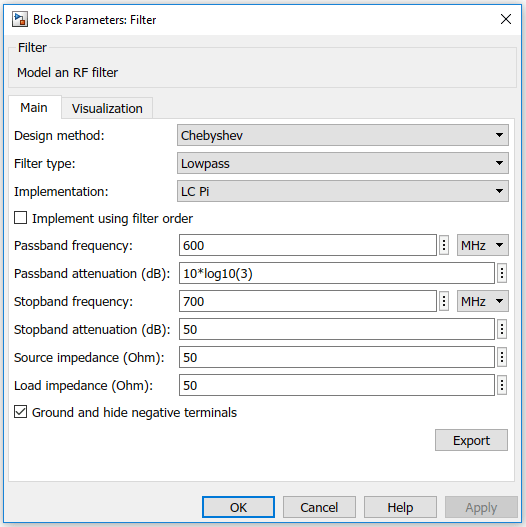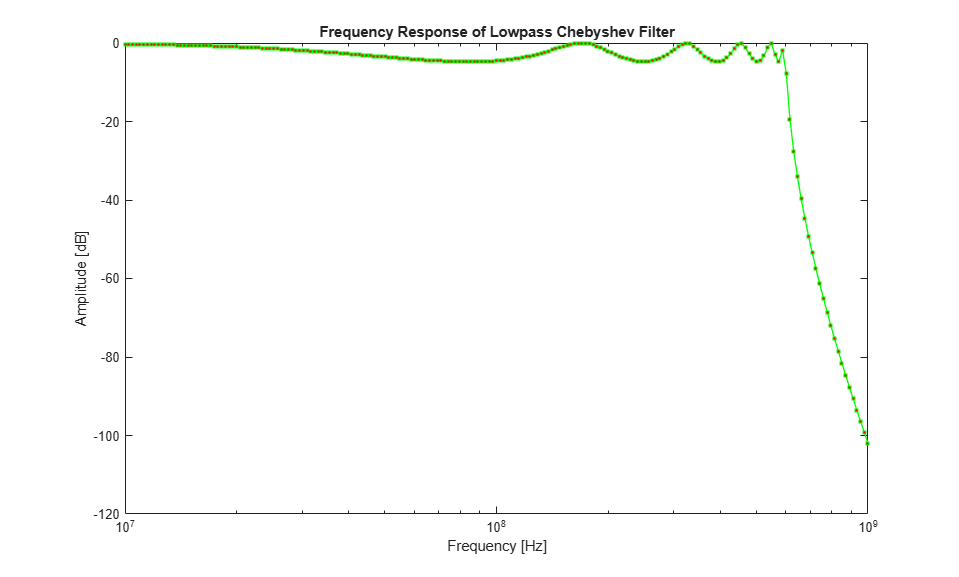# Frequency Response of Lowpass Chebyshev Filter

Use the `Filter` block to study the frequency response of a lowpass Chebyshev filter.

From the MATLAB command prompt, open the model.

```open_system('ex_simrf_filter_lowpass_cheby_resp') ```The `Constant` block sets the amplitude of the 201 carrier signals to ones (1, 201). The `Inport` block generates the 201 carrier frequencies for the mask value of logspace (7, 9, 201). Generate an 11th order lowpass LC Pi Chebyshev filter by setting appropriate block parameters in the `Filter` block.The output signal from the `Filter` block is fed into the `Outport` block. The `Outport` block is configured to give both magnitude and angle of the signal. The angle output is terminated using the `Terminator` block. The magnitude output is squared and converted to dB using `Math Function` and `dB Conversion` blocks.

To run the model, select Simulation > Run. You can also use the following command:

```sim('ex_simrf_filter_lowpass_cheby_resp') ```

The model creates an `Out` array in the MATLAB workspace. Since the simulation stop time is set to 0, the frequency response corresponds to the steady state solution.

To plot the frequency response, use the following commands in the MATLAB command window.

``` figure freq = logspace(7,9,201); h = semilogx(freq, Out, '-gs', 'LineWidth',1, 'MarkerSize',3, 'MarkerFaceColor','r'); xlabel('Frequency [Hz]'); ylabel('Amplitude [dB]'); title('Frequency Response of Lowpass Chebyshev Filter'); ```You can also use the Plot button in the Visualization tab of the `Filter` block parameters. Set the Frequency points to logspace (7, 9, 201) and X-axis scale to Logarithmic to achieve a similar plot.